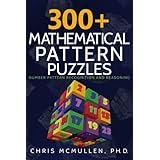# Five Math Puzzles (pattern recognition): Can You Solve Them?

## MATH PUZZLES

Here is a math puzzle challenge.

Hint: Each of the 5 patterns below has something in common.

Directions: See if you can figure out which numbers go in the blanks.

• 1, 2, 4, 6, 10, 12, 16, 18, 22, _, _
• 4, 6, 10, 14, 22, 26, 34, 38, _, _
• 3, 7, 13, 19, 29, 37, _, _
• 4, 9, 25, 49, 121, 169, 289, _, _
• 5, 8, 12, 18, 24, 30, 36, 42, 52, 60, 68, _, _

If you need help, you can find hints below.

But don’t scroll too far or you’ll run into the answers and explanations.

## PUZZLE HINT

Each pattern above has something in common.

They all involve prime numbers.

A prime number is only evenly divisible by two integers: 1 and itself.

For example, 7 is a prime number because the only integers that can multiply together to make 7 are 1 and 7.

In contrast, 6 isn’t a prime number because 2 x 3 = 6 (in addition to 1 x 6).

Here are the first several prime numbers.

2, 3, 5, 7, 11, 13, 17, 19, 23, 29, 31, 37

Each of the puzzles above relates to these prime numbers.

Here are the answers and explanations to the math puzzles:

• 28, 30. Explanation: Subtract 1 from each prime number: 2 – 1 = 1, 3 – 1 = 2, 5 – 1 = 4, 7 – 1 = 6, 11 – 1 = 10, etc.
• 46, 58. Explanation: Double each prime number: 2 x 2 = 4, 3 x 2 = 6, 5 x 2 = 10, 7 x 2 = 14, 11 x 2 = 22, etc.
• 43, 53. Explanation: Every other prime number: 3 (skip 5) 7 (skip 11) 13 (skip 17) 19 (skip 23) 29 etc.
• 361, 529. Explanation: Square each prime number: 2² = 4, 3² = 9, 5² = 25, 7² = 49, 11² = 121, etc.
• 78, 84. Explanation: Add consecutive prime numbers together: 2 + 3 = 5, 3 + 5 = 8, 5 + 8 = 13, 8 + 13 = 21, 13 + 21 = 34, etc.

## WANT MORE MATH PUZZLES?

One way is to follow my blog. I will post occasional math puzzles in the future.

Another way is to check out my newest book, 300+ Mathematical Pattern Puzzles.

It starts out easy and the level of challenge grows progressively so that puzzlers of all abilities can find many puzzles to enjoy.

A wide variety of topics are covered, including:

• visual patterns
• arithmetic
• repeating patterns
• Roman numerals
• Fibonacci sequence
• prime numbers
• arrays
• analogies
• and much moreThe cover was designed by Melissa Stevens at www.theillustratedauthor.net.

## CHRIS MCMULLEN, PH.D.

• 300+ Mathematical Pattern Puzzles
• Basic Linear Graphing Skills Practice Workbook
• Systems of Equations: Simultaneous, Substitution, Cramer’s Rule

Improve Your Math Fluency. Build fluency in:

• arithmetic
• long division
• fractions
• algebra
• trigonometry
• graphing
1.Sheldon Glover
•chrismcmullen